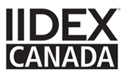×

×

It's easy to find the products and samples you need.

For advanced searches, start with "PRODUCTS" in the main navigation and find your category (e.g. wallcovering) or jump right in with a keyword search (above). Give us a call if you have any questions.

SEARCH EXAMPLES:
Product code #: DN2-CAP-08
Pattern name: Cappi
Brand: DeNovo
Type: Wallcovering, Wood, Paint, etc.

# INSPIRATION VIDEOS

Find the perfect wall design solution for your project.

Order a sample

Order a sample

Order a sample

Order a sample

Order a sample

Order a sample

Order a sample

Order a sample

Order a sample

Order a sample

Order a sample

Order a sample

Order a sample

Order a sample

Order a sample

Order a sample

Order a sample

Order a sample

Order a sample

Order a sample

Order a sample

Order a sample

Order a sample

Order a sample

Order a sample

Order a sample

Order a sample

Order a sample

Order a sample

Order a sample

Order a sample

Order a sample

Order a sample

Order a sample

Order a sample

Order a sample

Order a sample

Order a sample

Order a sample

Order a sample

Order a sample

Order a sample

Order a sample

Order a sample

Order a sample

Order a sample

Order a sample

Order a sample

Order a sample

Order a sample

Order a sample

Order a sample

Order a sample

Order a sample

Order a sample

Order a sample

Order a sample

Order a sample

## TABLE CORNER APPLICATION

Order a sample